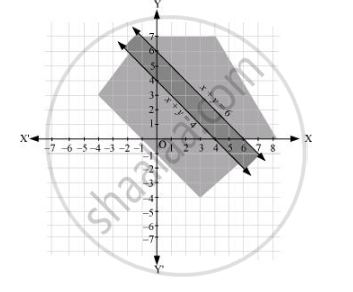# Solve the Following System of Inequalities Graphically: X + Y ≤ 6, X + Y ≥ 4 - Mathematics

Solve the following system of inequalities graphically: x + y ≤ 6, x + y ≥ 4

#### Solution

x + y ≤ 6 … (1)

x + y ≥ 4 … (2)

The graph of the lines, x + y = 6 and x + y = 4, are drawn in the figure below.

Inequality (1) represents the region below the line, x + y = 6 (including the line x + y = 6), and inequality (2) represents the region above the line, x + y = 4 (including the line x + y = 4).

Hence, the solution of the given system of linear inequalities is represented by the common shaded region including the points on the respective lines as follows.Concept: Solution of System of Linear Inequalities in Two Variables
Is there an error in this question or solution?
Chapter 6: Linear Inequalities - Exercise 6.3 [Page 129]

#### APPEARS IN

NCERT Class 11 Mathematics
Chapter 6 Linear Inequalities
Exercise 6.3 | Q 6 | Page 129

Share